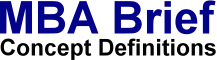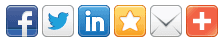# Hypothesis Testing

 Definition: Hypothesis Testing is a statistical method of reasoning and decision making using data from a scientific study. The question of interest is simplified into two competing claims / hypotheses between which we have a choice; the null hypothesis (H0) against the alternative hypothesis (H1). These two competing claims / hypotheses are not however treated on an equal basis: special consideration is given to the null hypothesis. Statistical hypothesis tests define a procedure which controls (fixes) the probability of incorrectly deciding that a default position (null hypothesis) is incorrect based on how likely it would be for a set of observations to occur if the null hypothesis were true.